# XOR In ExcelArticle byExcelMojo Team## What Is XOR Function In Excel?

The XOR in Excel returns TRUE for an odd number of TRUE inputs. And the function output will be FALSE if all the specified logical statements are false or the number of TRUE inputs is even.

The XOR function in Excel is an inbuilt Logical function whose output is the logical Exclusive OR of the given arguments.

For example, the below table shows the day-wise results of four tests. And the comments give the summary of the results for each test.

Suppose we have to show the day-wise comparison of the results for each test as a logical value in column F. Then, it is possible using XOR in Excel in the required target cells.

In the above XOR in Excel example, the function contains two logical statements. And it returns TRUE if only one logical statement holds. Otherwise, it returns FALSE.

Likewise, we can apply the function XOR in Excel to compare a maximum of 254 logical conditions.

###### Key Takeaways
• The XOR in Excel is a Logical function.
• The syntax of XOR() is =XOR(logical1,[logical2],…) where logical1 is the logical value we require to test and logical2 indicates the other values we require to test.
• The XOR() accepts one logical value as mandatory input, while the remaining arguments are optional.
• The XOR() gives excellent results when used with other Excel functions such as IF. And when using XOR() in Excel VBA, the expression will be: expression1 Xor expression2 where expression1 and expression2 are the two logical statements we need to evaluate using the XOR().

### XOR() Excel Formula

The syntax of XOR Formula in Excel is:

where,

• logical1: It is the logical value we require to test.
• logical2, ….: The following logical values we require to test.

While the first argument of the function XOR in Excel is mandatory, the subsequent arguments are optional.

Also, below are a few aspects we must consider to avoid the scenario of the function XOR in Excel not working.

• The arguments we provide to the XOR() should evaluate to logical values. They can be TRUE or FALSE, references to cells or arrays containing logical values.
• The XOR() can test up to 254 logical statements.
• If the XOR() arguments contain text values or blank cells, the function ignores such cells during the calculation.
• If the given data range does not contain logical values, the function XOR in Excel throws the #VALUE! error.

### How To Use XOR Excel Function?

The steps to use the function XOR in Excel are:

1. First, confirm the input data evaluates to logical values.
2. Then, select the target cell and enter the function XOR in Excel.
3. Finally, press Enter to view the XOR() output.

The below illustration explains the XOR in Excel meaning and the steps mentioned above.

Consider the below table. It contains two sets of values in columns A and B, respectively.

Suppose we aim to supply the data points of the two data sets as the arguments to the XOR() and display the output in column C. Then, here are the steps:

1. Select the target cell C2, enter the XOR() provided in the Formula Bar in the below image, and press Enter.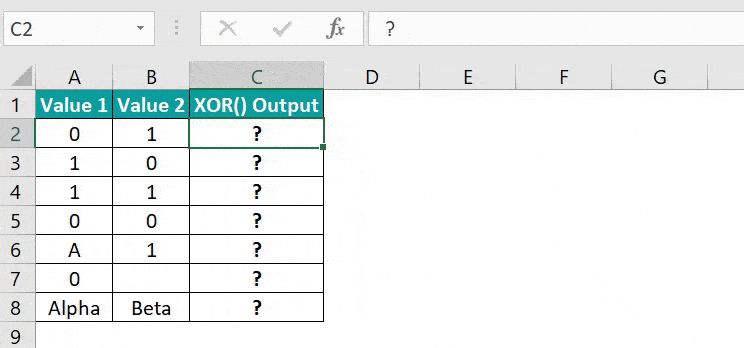Alternatively, we can apply the XOR() using the option available in the Formulas tab. Select the target cell C2 and click FormulasLogicalXOR to open the Function Arguments window.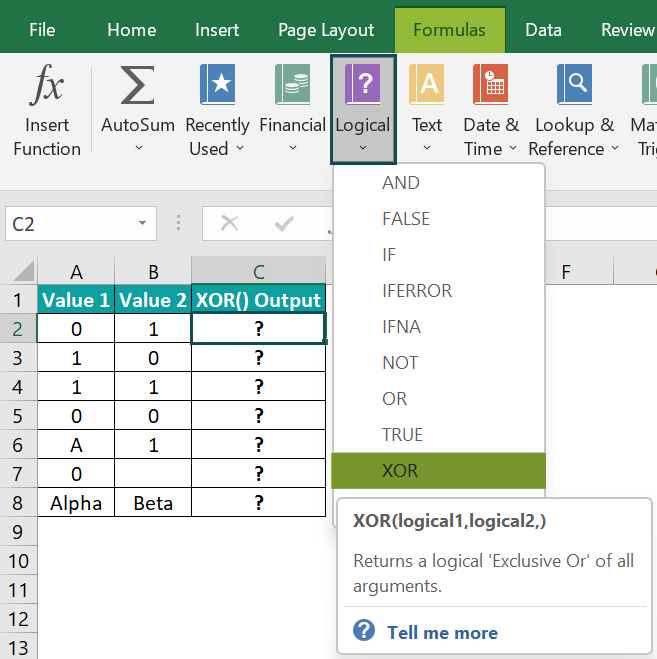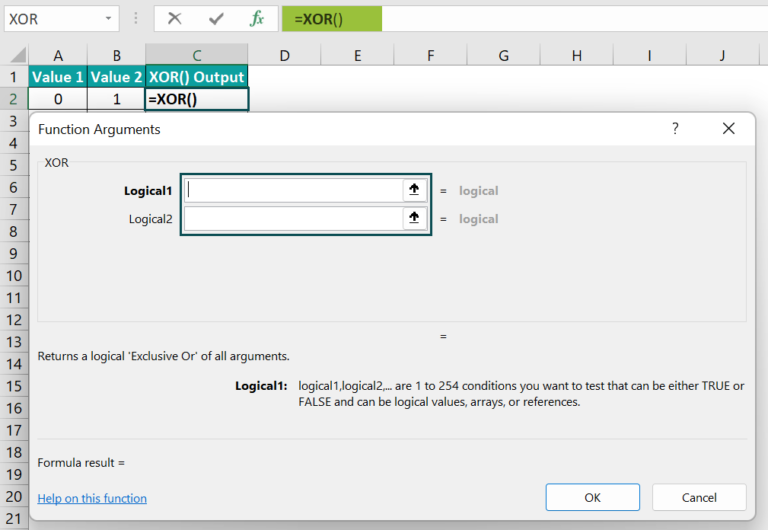Then, enter the two argument values in the Function Arguments window.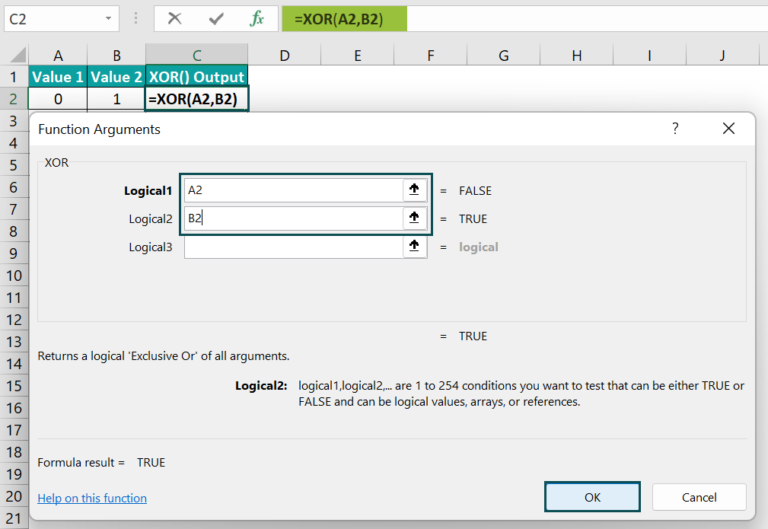And once we click OK in the Function Arguments window, the XOR() in the target cell C2 gets executed.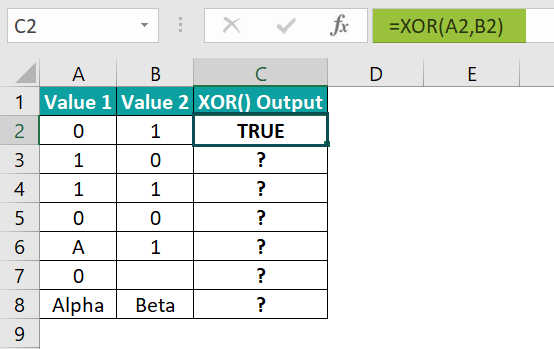Please Note: When we click the last available field in the Function Arguments window, the field to enter the next logical value appears. In the above illustration, the total number of arguments is two. And thus, we only need to enter the values in the Logical1 and Logical2 fields.

2. Drag the fill handle downwards to copy the formula in the cell range C3:C8.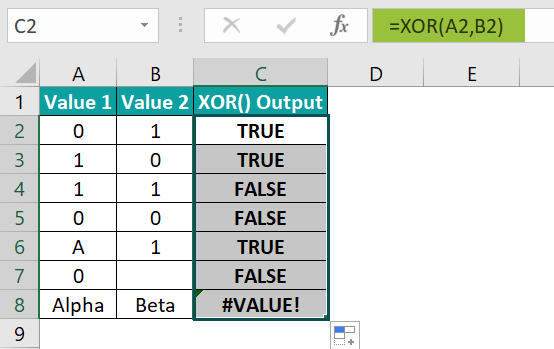In the above XOR in Excel example, the values 1 and 0 interpret as TRUE and FALSE, respectively. So, the XOR() in cells C2:C3 returns TRUE as one out of the two provided logical statements is true. On the other hand, in the target cell C4 formula, the number of TRUE inputs is even, and in the cell C5 formula, all the logical statements are false. Hence the XOR() in the cell range C4:C5 returns FALSE.

In the case of row 6, the first argument is a text value, which the function ignores. But the second argument interprets as TRUE. So, the XOR() output in cell C6 is TRUE. Likewise, in row 7, the XOR() ignores the empty cell and returns FALSE, based on the first argument value 0.

And in row 8, both the arguments are neither logical values nor do they evaluate to logical values. So, it becomes a case of the function XOR in Excel not working, and thus, we get the #VALUE! error as the XOR() output in the target cell C8.

### Examples

The following examples will explain the XOR in Excel meaning more clearly.

#### Example #1

Let us see how the function XOR in Excel behaves when there are more than two arguments.

The following table contains a list of conditions.

And suppose the conditions in each row in the cell range A2:E6 are the arguments for the XOR(), and we require to display the output in column F. Then here is how using XOR in Excel option, we can update the required target cells.

Step 1: Select the target cell F2, enter the XOR(), and press Enter.

=XOR(10>20,10=20,TRUE,1,15)

In the above formula, the first two arguments are logical statements that evaluate to logical values, with both conditions being FALSE. And the last three values interpret as TRUE. So, as there are an odd number of TRUE values, the function XOR in Excel returns TRUE.

Step 2: Select the target cell F3, enter the XOR(), and press Enter.

=XOR(9<=23,1>-45,TRUE, FALSE,0)

Here, the same logic explained for the XOR() in row 2 applies. The first three logical values evaluate to TRUE and the last two as FALSE. So, the XOR() returns TRUE as the output.

Step 3: Select the target cell F4, enter the XOR(), and press Enter.

=XOR(30=30,B4:E4)

In the above XOR formula, the second argument is an array that evaluates to a logical value since it contains FALSE values. So, here the first argument value is TRUE, while the second argument value is FALSE. And thus, the XOR() output is TRUE.

Step 4: Select the target cell F5, enter the XOR(), and press Enter.

=XOR(A5:C5,10<>20,90>60)

In the above formula, all argument values are TRUE. And as the number of TRUE values is odd, the XOR() output is TRUE.

Step 5: Select the target cell F6, enter the XOR(), and press Enter.

=XOR(A6:E6)

In this case, all arguments are logical values. And so, we enter them as an array range while supplying the argument values to the XOR(). And since the number of TRUE values is even, the function returns FALSE.

#### Example #2

This example explains how to apply the XOR with IF Excel Function.

The below table shows the results of two rounds in a Quiz program involving five teams.

The teams that won Round 1 and Round 2 do not have to play Round 3. And those that lost both rounds cannot appear for the third round. But, on the other hand, the teams that won one of the rounds need to play Round 3.

We can use the function XOR in Excel within the IF() to update the required data in the target cell range D2:D6 while adhering to the above-mentioned conditions.

Step 1: Select the target cell D2, enter the following IF() with the XOR(), and press Enter.

=IF(XOR(B2=”WON”,C2=”WON”),”YES”,”NO”)

Step 2: Drag the fill handle downwards to copy the formula in cell range D3:D6.

We shall consider the formula in the target cell D6 to understand how the formula works. First, the XOR() checks the two given logical statements. While the first condition is false, the second one holds. So, as the number of TRUE values is odd, 1, the XOR() returns TRUE as the output. And thus, the IF condition holds, and the IF() returns YES as the output in the target cell D6.

#### Example #3

We shall now see how to apply the function XOR in Excel through VBA Editor.

Suppose the given data includes three values, value1, value2, value3, and the specific conditions involving these values we need to check.

And we require to display the output in cell range B7:B9. So then, here is how we can apply the function XOR in Excel VBA.

Step 1: With the worksheet containing the above table, press the excel keyboard shortcut keys Alt + F11 to open the VBA Editor.

Step 2: Choose the required VBAProject from the menu and click InsertModule to open the Module1 window.

Step 3: Enter the VBA code in the Module1 window to apply the XOR().

Sub XOR_fn()

Dim value1 As Integer

Dim value2 As Integer

Dim value3 As Integer

Value1 = 100

Value2 = 50

Value3 = 25

Range(“B7”) = (value1 > value2) Xor (value2 > value3)

Range(“B8”) = (value2 > value1) Xor (value2 > value3)

Range(“B9”) = (value2 > value1) Xor (value3 > value2)

End Sub

Step 4: Click on the Run Sub/UserForm icon on the top menu to run the code.

Step 5: Open the worksheet to view the output in the target cells.

In the above example, the VBA code contains the XOR() in three command lines. And it compares two logical expressions in each line. So, for example, the output in the target cell B8 is TRUE, as the first expression, value2>value1, is false, and the second one, value2>value3, is true.

### Important Things To Note

• The function XOR in Excel can accept up to 254 arguments.
• The XOR() arguments can be TRUE or FALSE, references to cells or arrays containing logical values, or inputs that evaluate to logical values.
• The XOR function ignores text values and blank cells.
• If the given input to the XOR() does not contain any logical values, the function returns the #VALUE! error.
• The function XOR in Excel returns the Exclusive OR of the specified logical values. The function output is TRUE if the number of logical values evaluating to TRUE is odd. And the function output is FALSE if the number of logical values evaluating to TRUE is even or all the logical values are FALSE.

1. Where is the XOR function in Excel?

The XOR function is in the Formulas tab. We can click FormulasLogical XOR to access it.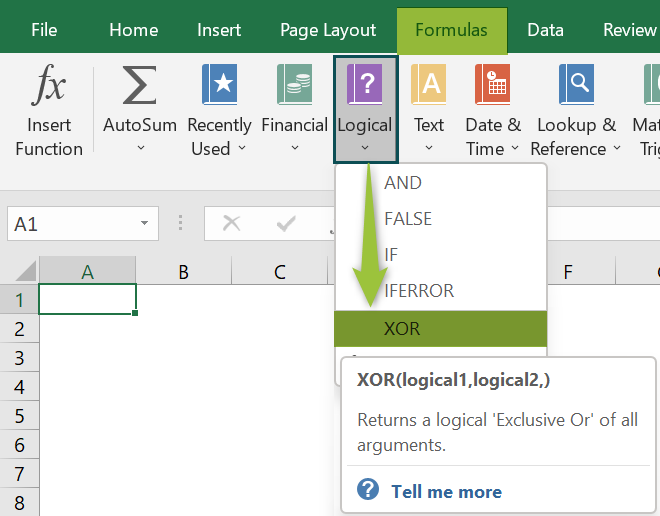2. Why is my XOR function in Excel not working?

The XOR function in Excel may not work because the given arguments
Do not contain any logical values.
Do not evaluate to logical values.
Are empty cells or text values.

3. What is the difference between the functions OR and XOR in Excel?

The difference between the functions OR and XOR in Excel is that the OR() output is TRUE if any supplied input is TRUE. On the other hand, the XOR() returns the Exclusive OR of the provided arguments. It returns TRUE for an odd number of TRUE inputs and FALSE for an even number of TRUE inputs or when all inputs are FALSE.

For example, consider the following table. It shows two logical values, Logical1 and Logical2.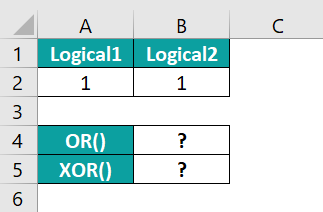Here is how the OR() and XOR() work. And we shall display the output in the target cells B4:B5.

Step 1: Select the target cell B4, enter the following OR(), and press Enter.
=OR(A2:B2)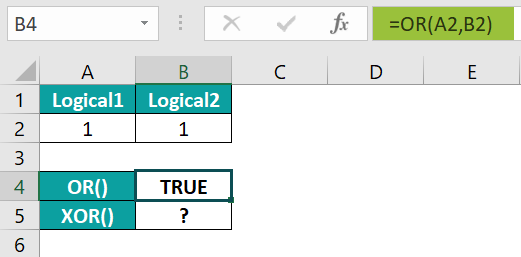Step 2: Select the target B4, enter the following XOR(), and press Enter.
=XOR(A2:B2)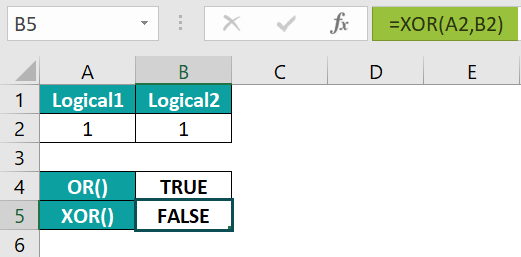Thus, the result shows that the OR() returns TRUE as at least one of the two inputs is TRUE. On the other hand, as the number of TRUE inputs in the XOR() is even, the function output is FALSE.